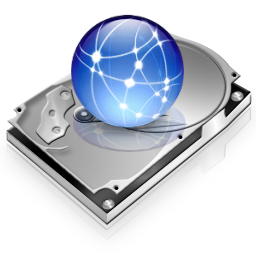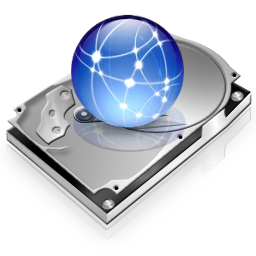## Steganography with Clojure - Hiding Text in Images

Steganography is the process of hiding data in other data so no one apart from the sender and the receiver knows the existence or transmission of the message. It allows us to send a message within a seemingly unimportant message or something that does not attract attention.

Steganography has been used throughout the history, some old school methods include,

• Greeks and wax covered tablets
• Histiaeus and the shaved head
• Invisible inks in WWII
• Microdots

This post will cover hiding textual data in images using LSB (Least Significant Bit) technique. Each pixel in an image is a 32 bit int, split into 8 bit values representing alpha, red, green, blue.

0xAARRGGBB


Changing least significant bit in each of these four values would allow minor variations in color and it should be unnoticable to the naked eye, even when noticed it can easily be mistaken for flaws in the quality of the picture. So by changing last bit of all four values we can encode 4 bits of data per pixel. (Not all image formats support alpha for those you can encode 3 bits per pixel.)

(ns steganography
(:use clojure.contrib.seq-utils)
(:import (javax.imageio ImageIO)
(java.io File)))

(defn bits [n]
(reverse (map #(bit-and (bit-shift-right n %) 1) (range 8))))


Given a byte bits will return a sequence of bits that represent that byte,

steganography=> (bits (int \C))
(0 1 0 0 0 0 1 1)


numb reverses the process given a sequence of bits, you get the original byte,

(defn numb [bits]
(BigInteger. (apply str bits) 2))

steganography=> (char (numb (bits (int \C))))
\C


Using set-lsb we will encode one bit per a r g b value, given a byte and one bit from the data, we set the LSB to the bit given,

(defn set-lsb [bits bit]
(concat (take 7 bits) [bit]))

steganography=> (set-lsb (bits 255) 0)
(1 1 1 1 1 1 1 1) => (1 1 1 1 1 1 1 0)


We take the string we want to encode, pad it with ";" which will indicate we have reached the end of our message while decoding, then turn it into a sequence of bits,

(defn string-to-bits [msg]
(flatten (map #(bits %) (.getBytes (str msg ";")))))

steganography=> (string-to-bits "cb")
(0 1 1 0 0 0 1 1 0 1 1 0 0 0 1 0 0 0 1 1 1 0 1 1)


Next using this bit sequence we created, we match every four bits to a coordinate,

(defn get-argb [img cord]
(let [[x y] cord
clr (.getRGB img x y)]
[(bit-and (bit-shift-right clr 24) 0xff)
(bit-and (bit-shift-right clr 16) 0xff)
(bit-and (bit-shift-right clr 8) 0xff)
(bit-and clr 0xff)]))

(defn set-argb [img cord color]
(let [[x y] cord
[a r g b] color
c (bit-or (bit-shift-left a 24)
(bit-or (bit-shift-left r 16)
(bit-or (bit-shift-left g 8) b)))]
(.setRGB img x y c)))

(defn match-bits-coords [bits img]
(partition 2
(interleave (partition 4 bits)
(take (/ (count bits) 4)
(for [x (range (.getWidth img))
y (range (.getHeight img))] [x y])))))

steganography=> (match-bits-coords (string-to-bits "c")
(((0 1 1 0) [0 0]) ((0 0 1 1) [0 1])
((0 0 1 1) [0 2]) ((1 0 1 1) [0 3]))


We iterate over this bit coordinate sequence, for each pixel, we retrieve its argb value, match each a r g b vals with a bit, then encode it using set-lsb and set this new color we calculated for the pixel,

(defn set-pixels [img d]
(doseq [[data cord] d]
(let [color-bit (partition 2 (interleave (get-argb img cord) data))
color (map #(let [[n b] %]
(numb (set-lsb (bits n) b))) color-bit)]
(set-argb img cord color))))


In order to encode data, we read the image, match bits to coordinates, iterate through the pixels calculating and setting new colors and finally writing the image,

(defn encode [fname msg]
data (match-bits-coords (string-to-bits msg) img)]
(set-pixels img data)
(ImageIO/write img "png" (File. (str "encoded_" fname)))))

(encode "drive.png" "Attack At Down!!")


Extracting data we encoded is much simpler,

(defn get-pixels [img]
(map #(get-argb img %) (for [x (range (.getWidth img))
y (range (.getHeight img))] [x y])))

steganography=> (take 3 (get-pixels (ImageIO/read (File. "encoded_drive.png"))))
([0 255 254 254] [0 254 254 255] [0 255 255 255])


First build a sequence of argb values for each pixel,

(defn split-lsb [data]
(map #(last (bits %)) data))


after flattening this sequence, we extract least significant bit from each byte giving us a sequence of 0's and 1's. Our original string as a bit string,

(defn decode [fname]
to-char #(char (numb (first %)))]
(loop [bytes (partition 8 (split-lsb (flatten (get-pixels img))))
msg (str)]
(if (= (to-char bytes) \;)
msg
(recur (rest bytes) (str msg (to-char bytes)))))))

(decode "encoded_drive.png")


Now all we have to do is partition that sequence into groups of 8, each representing a char. We just keep casting bits into a char until we read ";" which denotes we have reached the end of our message. Okay, enough typing let's see it in action, assuming we want to encode "Attack At Down!!".

Image before steganography,steganography=> (encode "drive.png" "Attack At Down!!")
steganography=> (decode "encoded_drive.png")
"Attack At Down!!"


Image after steganography,You are not limited to encoding text in images, you can embed images within images, although I used 4 bits per pixel if you think you can get away with more degradation in quality you can embed more bits per pixel.